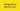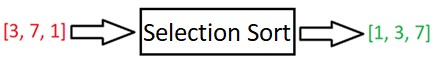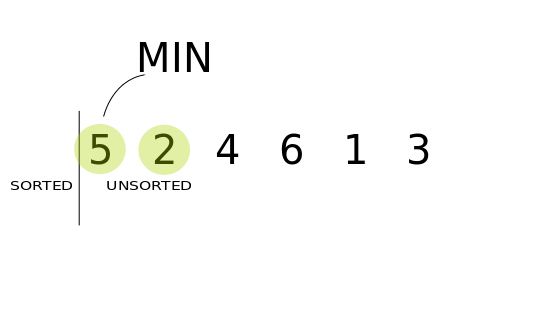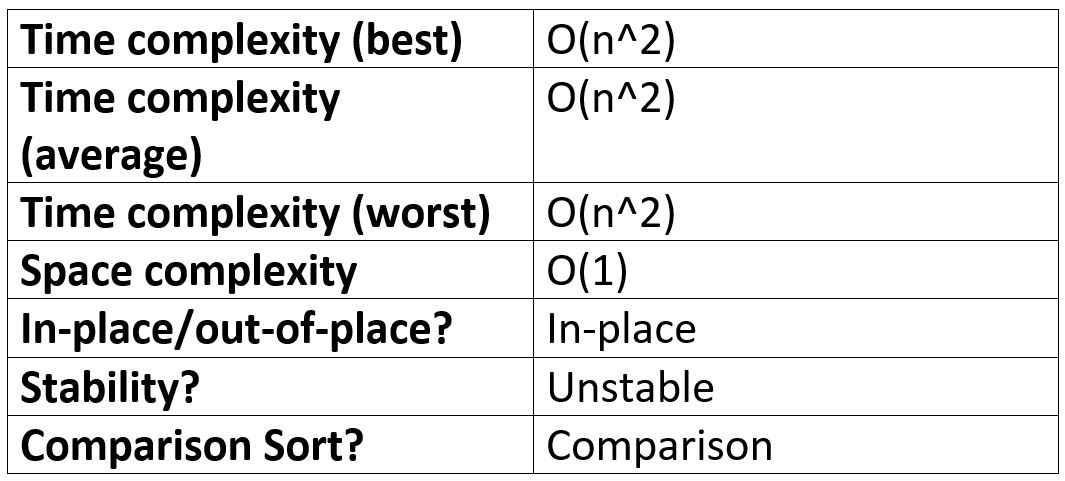# Selection Sort - JavaScript

-03 August 2021## Intro

Selection Sort is one of the more straight-forward sorting algorithms and is so a great sorting algorithm to introduce to beginners. And whilst there exist more efficient sorting algorithms, it can still outperform them in certain use cases.

• what is the Selection Sort algorithm?
• the logic of Selection Sort.
• how to implement Selection Sort in JavaScript.
• the performance of Selection Sort.
• when and when not to use Selection Sort.

Disclosure: I’m always looking for things I think my readers will value. This article contains some affilate links to products that I have used and found helpful. If you purchase these, then I may see a share of the revenue. This comes at no extra cost to you.

## What is the Selection Sort Algorithm?

Selection Sort is a sorting algorithm, meaning it takes in an array and puts it in order:Selection Sort is an in-place, unstable and comparison-type algorithm.

In-place means that when transforming the input, no auxiliary data structure is used (apart from a small amount of extra storage for auxiliary variables). In simple terms, it usually just means that the input is overwritten (via swapping or replacement) by the output as the algorithm executes. The advantage of in-place algorithms is that they take up less space in memory.

Unstable means that two elements with equal values could appear in different order in the sorted output compared with how they appeared in the unsorted input array.

For example, [“Andrew”, “Betty”, “Adam”] could be sorted to [“Adam”, “Andrew”, “Betty”] using an unstable algorithm – notice how “Adam” and “Andrew” changed relative order despite having the same first letter; this wouldn’t happen if we used a stable algorithm, like Insertion Sort.

And finally, a comparison sort is a sorting algorithm that only reads the list of elements through a single abstract comparison operation (usually a “less than” or “equal to”) that determines which of the two elements should occur first in the final sorted output array.

Check out my In-place, stable, comparison article if you don’t quite understand the above, or don’t know why it’s important to know these properties of an algorithm.

## Selection Sort step by step

Selection sort works by going up an array and selecting the minimum value. The minimum value is then moved to the beginning of the array. The left side of the array becomes more sorted at the end of each pass through the array, until the whole array is sorted.

It’s similar to Bubble Sort, but instead of the largest values “bubbling” to the top, the smallest values are selected and placed at the beginning.To get Selection Sort’s logic clear in our heads, let’s walk through the steps in the gif above:

1. Pass in the array [5, 2, 4, 6, 1, 3] to selection sort.
2. Start at element 1 (5) and set it as the minimum value.
3. Compare element 1 (5) with element 2 (2). 2 is less than 5, so set 2 as the new minimum value.
4. Move up to element 3 (4).
5. 4 < 2? No, so move up to element 4 (6).
6. 6 < 2? No, so move up to element 5 (1).
7. 1 < 2? Yes, so set 1 as the new minimum.
8. Move up to element 6 (3).
9. 3 < 1? No.
10. We’ve reached the end of the array so swap element 1 (5) with our minimum element – element 5 (1) => [1, 2, 4, 6, 5, 3]
11. Element 1 is now sorted. We now start at element 2, pass through the array to find the minimum, and put it in second place. This process is repeated until we’ve checked that every element is in its correct place.

## Selection Sort - JavaScript

First, study the code below:

`function selectionSort(arr) {  for (let i = 0; i < arr.length; i++) {    let lowest = i    for (let j = i + 1; j < arr.length; j++) {      if (arr[j] < arr[lowest]) {        lowest = j      }    }    if (lowest !== i) {      // Swap      ;[arr[i], arr[lowest]] = [arr[lowest], arr[i]]    }  }  return arr}console.log(selectionSort([3, 5, 1, 2])) // [1, 2, 3, 5]`

Let’s now go through how this is working:

1. First, we create an outer loop to go over each element once, and store the first element as the smallest value we’ve seen so far in a variable called “lowest”.
2. We then create an inner for-loop to go over each element in the array, starting from i + 1.
3. Inside this inner-loop, we check if we the current value is less than our current lowest. If so, we update our lowest to the index of that value.
4. After the inner-loop has reached the end of the array, we check to see if the lowest ever got changed; if so, we swap it with arr[i].
5. We then go back to outer loop, increment i by 1 (element 2) and repeat the process until each element in the array has been checked.

## Time complexity of Selection Sort in all cases

We will now discuss the time complexity of Selection Sort in best, average and worst case. If you’re not familiar with Big O Notation, then check out: Big O Notation in JavaScript | The Ultimate Beginners Guide with Examples. Big O is important and can prevent you from writing an inefficient algorithm at the wrong time!

### Best case time complexity of Selection Sort

If the array is nearly sorted, e.g. [2, 1, 3, 4], or is sorted, then for each element in the array, Selection Sort will run through the array looking for the minimum, even if no swaps are necessary.

Even if the array is sorted, there will be roughly n * n comparisons (but no swaps), where n is the number of elements in the input array.

So, at best-case we’ll have O(n^2) comparisons and O(1) swaps.

This means that overall, Selection Sort has a very bad best-case run time of O(n^2) – quadratic time complexity.

### Worst case time complexity of Selection Sort

If the array is in reverse order, e.g. [4, 3, 2, 1], then it will take just as many comparisons as if the array was almost sorted – O(n^2). There will also be O(n) swaps. Total operations will therefore be O(n + n^2).

As the n^2 has the largest contribution, the overall Big O of Selection Sort is O(n^2) – quadratic time complexity.

### Average case time complexity of Selection Sort

Selection Sort will run at quadratic time no matter what order the input array is in; the number of comparisons will always be the same for a given length of array. Although, if array is almost sorted, then there will be less swaps.

So, the average case time complexity of Selection Sort, is O(n^2).

## Selection Sort space complexity

Selection Sort is an in-place algorithm, meaning it does not need any extra space, and produces an output in the same memory that contains the data by transforming the input “in-place”.

No extra data structures - apart from a few small constant-space variables: `i`, `j`, and `lowest` in our case - need to be stored in memory.

Therefore, Selection sort has a space complexity of O(1) – constant space complexity.

## Selection Sort performance summary table## When to use Selection Sort

One thing which distinguishes selection sort from other sorting algorithms is that it makes the minimum possible number of swaps: n − 1 in the worst case.

This is a lot better than Bubble Sort which potentially swaps at every comparison - n^2. This could be useful if, for some reason, you were worried about writing to memory a lot, but this is rarely a problem.

It can be seen as an advantage in some situations that Selection Sort will always perform the same no matter what the order of the array; but usually, this is a disadvantage: it’s usually better if an algorithm varies a lot in its performance if it means it performs the sort quicker when the array is almost sorted. For these reasons, Insertion Sort is often a better choice.

For larger arrays, Selection Sort is greatly outperformed by O(n log(n)) divide-and-conquer algorithms such as Merge Sort.

However, Selection Sort and Insertion Sort are both typically faster for small arrays (< 20 elements), so it could be a useful optimization to check the size of the input array and perform Selection or Insertion Sort if the array is small, and a more efficient algorithm, like Merge Sort, if the array is larger.

To summarise: Selection Sort is a simple, but inefficient Sorting algorithm. It outperforms Quick Sort and Merge Sort if the array is small, but so does Insertion Sort, and Insertion Sort is usually more effective in these cases.

## If you Want to Master Algorithms...

If you want to further your knowledge of algorithms and data structures, check out: JavaScript Algorithms and Data Structures Masterclass by Colt Steele. It’s the best Udemy course I’ve ever taken 👌.

If you enjoyed this article, you can say thanks by subscribing to my YouTube channel or by signing up to my blog to be notified of new posts 🙏

Also, feel free to connect with me on Twitter!